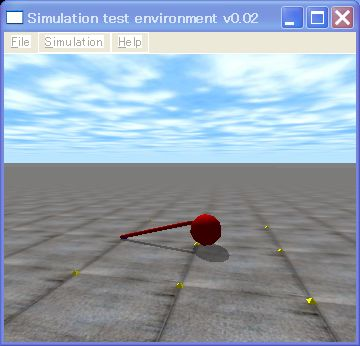# ODE Tutorial 8: Joint MotionODE (Open Dynamics Engine) lecture time it is 8th. This time the waist joint which the last time was made (the hip joint) it will move.

It moves the joint with procedure below.

1. The movable limits of the joint are set
dJointSetHingeParam (the lower limit of dJointID, dParamLoStop and movable limits);
dJointSetHingeParam (the upper limit of dJointID, dParamHiStop and movable limits);
2. Target angular velocity of the joint largest torque because it actualizes that is set
dJointSetHingeParam (dJointID, dParamVel and  target angular velocity);
dJointSetHingeParam (dJointID and dParamFMax, largest torque)

With ODE being there is no API which appoints the target angle of the joint directly, in order to keep having joint angle in target angle, until you acquire present joint angle with dJointGetHingeAngle, become that angle using dJointSetHingeParam, when it gives angular velocity to the joint and becomes target angle the method is used of designating angular velocity as 0.

So, source code is shown below. Object the body (torso), on thign, it has modified the difference with the last time in 1 foot robot MonoBot which has the below the knee. Degree of freedom the waist joint (hip joint) 1, the knee joint (knee joint) total 2 of 1 it has become degree of freedom, but this time just the waist joint moves.

[code]
// sample4.cpp by Kosei Demura

#ifdef dDOUBLE
#define dsDrawSphere dsDrawSphereD
#define dsDrawCapsule dsDrawCapsuleD
#endif

MyLink torso and leg [ 2 ];
dJointID joint [ 2 ];

enum jointNo {
HIP,
KNEE
};

// Control of joint
void control ()
{
double velCoeff = 1.0; // Proportional constant
double p; // Present joint angle
static double angle = 0.5 * M_PI;

p = dJointGetHingeAngle (joint [ HIP ]); // acquisition of present joint angle
dReal z = angle – p; // As for angle target angle
if (p > 0.24 * M_PI) angle = – 0.25 * M_PI;
else {
if (p < – 0.24 * M_PI) angle = 0.25 * M_PI;
}
dJointSetHingeParam (joint [ HIP ], dParamVel and velCoeff*z); // Setting of target angular velocity
dJointSetHingeParam (joint [ HIP ], dParamFMax, 100); // Setting of max torque
}

static void simLoop (int pause)
{
const dReal *pos1, *R1, *pos2, *R2;

time++;
control (); // Control of joint
dSpaceCollide (space,0, &nearCallback);
dWorldStep (world,0.01);
dJointGroupEmpty (contactgroup);
dsSetColor (1.0,0.0,0.0);

// Drawing the draw a torso body
pos1 = dBodyGetPosition (torso.body);
R1 = dBodyGetRotation (torso.body);

// Draw legs
for (int i = 0; I< 2; I++) {
pos2 = dBodyGetPosition (leg [ i ] body);
R2 = dBodyGetRotation (leg [ i ] body);
dsDrawCapsule (pos2, R2, leg [ i ] length, leg [ i ] radius);
}
}

// Formation of Create a monobot one foot robot
void createMonoBot () {
dMass m1;
DReal x0 = 0.0, y0 = 0.0, z0 = 2.5;

// Torso body
torso.mass = 10.0;
torso.body = dBodyCreate (world);
dMassSetZero (&m1);
dBodySetMass (torso.body and &m1);
dBodySetPosition (torso.body and x0, y0, z0);
torso.geom = dCreateSphere (space and torso.radius);
dGeomSetBody (torso.geom and torso.body);

for (int i = 0; I < 2; I++) {
leg [ i ] radius = 0.025; Leg [ i ] length = 0.5; Leg [ i ] mass = 1.0;
leg [ i ] body = dBodyCreate (world);
dMassSetZero (&m1);
dMassSetCapsuleTotal (&m1, leg [ i ] mass,3, leg [ i ] radius, leg [ i ] length);
dBodySetMass (leg [ i ] body, &m1);
dBodySetPosition (leg [ i ] body, x0, y0, z0 -torso.radius
– 0.5 * (2 * i + 1) *leg [ i ] length);
leg [ i ] geom = dCreateCapsule (space and leg [ i ] radius, leg [ i ] length);
dGeomSetBody (leg [ i ] geom, leg [ i ] body);
}

joint [ HIP ] = dJointCreateHinge (world, 0);
dJointAttach (joint [ HIP ], torso.body and leg [ 0 ] body);
dJointSetHingeAnchor (joint [ HIP ], x0, y0, z0 – torso.radius);
dJointSetHingeAxis (joint [ HIP ], 1, 0, 0);
dJointSetHingeParam (joint [ HIP ], dParamLoStop, -0.25 * M_PI); // The movable limits setting lower limit
dJointSetHingeParam (joint [ HIP ], dParamHiStop, 0.25 * M_PI); // As for upper limit unit radian

// Knee joint knee joint
joint [ KNEE ] = dJointCreateHinge (world, 0);
dJointAttach (joint [ KNEE ], leg [ 0 ] body, leg [ 1 ] body);
dJointSetHingeAnchor (joint [ KNEE ], x0, y0, z0 – torso.radius – leg [ 0 ] length);
dJointSetHingeAxis (joint [ KNEE ], 1, 0, 0);
dJointSetHingeParam (joint [ KNEE ], dParamLoStop, 0.0 * M_PI); // Movable limits
dJointSetHingeParam (joint [ KNEE ], dParamHiStop, 0.0 * M_PI); // As for M_PI piπ(pie)

}

int main (int argc, char *argv[])
{
setDrawStuff ();

world = dWorldCreate ();
space = dHashSpaceCreate (0);
contactgroup = dJointGroupCreate (0);

dWorldSetGravity (world,0,0, -9.8);
ground = dCreatePlane (space,0,0,1,0);
createMonoBot (); // Formation of one foot robot
dsSimulationLoop (argc and argv,352,288, &fn);
dWorldDestroy (world);
return 0;
}
[/code]

The function control which is moving joint set point and presently being proportionate to the difference of value, has become the P control which it controls. As for parameter dParamVel of the joint at target angular velocity, as for dParamFMax it is the largest torque which can show the joint because that angular velocity is actualized. When this value is set to 0, setting target angular velocity, torque 0 being to be, it does not move with the joint.

To be continued.

english
スポンサーリンク
demura.netをフォローするdemura.net

## コメント

1. demu より:

Hi ranu wijoyo,

Thank you very much for your comment.